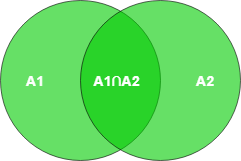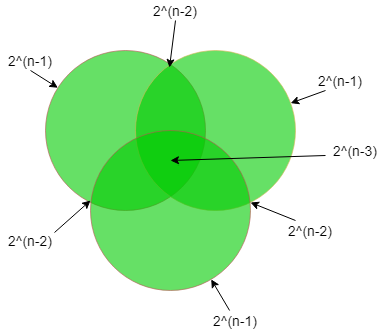# Number of Possible Super Keys in DBMS

• Difficulty Level : Medium
• Last Updated : 25 Oct, 2021

Prerequisite – Relational Model Introduction and Codd Rules

Any subset of attributes of a table that can uniquely identify all the tuples of that table is known as a Super key. It’s different from the primary and candidate keys in the sense that only the minimal superkeys are the candidate/primary keys.

Attention reader! Don’t stop learning now.  Practice GATE exam well before the actual exam with the subject-wise and overall quizzes available in GATE Test Series Course.

Learn all GATE CS concepts with Free Live Classes on our youtube channel.

This means that from a super key when we remove all the attributes that are unnecessary for its uniqueness, only then it becomes a primary/candidate key. So, in essence, all primary/candidate keys are super keys but not all super keys are primary/candidate keys. By the formal definition of a Relation(Table), we know that the tuples of a relation are all unique. So, the set of all attributes itself is a super key.

Counting the possible number of super keys for a table is a common question for GATE. The examples below will demonstrate all possible types of questions on this topic.

• Example-1 : Let a Relation R have attributes {a1,a2,a3} and a1 is the candidate key. Then how many super keys are possible?

Here, any superset of a1 is the super key.
Super keys are = {a1, a1 a2, a1 a3, a1 a2 a3}
Thus we see that 4 Super keys are possible in this case.

In general, if we have ‘N’ attributes with one candidate key then the number of possible superkeys is 2(N – 1)

• Example-2 : Let a Relation R have attributes {a1, a2, a3,…,an}. Find Super key of R.
Maximum Super keys = 2n – 1.
If each attribute of relation is candidate key.

• Example-3: Let a Relation R have attributes {a1, a2, a3,…, an} and the candidate key is “a1 a2 a3” then the possible number of super keys?

Following the previous formula, we have 3 attributes instead of one. So, here the number of possible super keys is 2(N-3)

• Example-4: Let a Relation R have attributes {a1, a2, a3,…, an} and the candidate keys are “a1”, “a2” then the possible number of super keys?

This problem now is slightly different since we now have two different candidate keys instead of only one. Tackling problems like these is shown in the diagram below:• → |A1 ∪ A2| = |A1| + |A2| – |A1 ∩ A2|

= (super keys possible with candidate key A1) + (super keys possible with candidate key A2) – (common superkeys from both A1 and A2)

= 2(n-1) + 2(n-1) – 2(n-2)

• Example-5: Let a Relation R have attributes {a1, a2, a3,…, an} and the candidate keys are “a1”, “a2 a3” then the possible number of super keys?

Super keys of (a1) + Super keys of (a2 a3) – Super keys of (a1 a2 a3)
⇒ 2(n – 1) + 2(n – 2) – 2(n – 3)

• Example-6: Let a Relation R have attributes {a1, a2, a3,…, an} and the candidate keys are “a1 a2”, “a3 a4” then the possible number of super keys?

Super keys of(a1 a2) + Super keys of(a3 a4) – Super keys of(a1 a2 a3 a4)
⇒ 2(n – 2) + 2(n – 2) – 2(n – 4)

• Example-7: Let a Relation R have attributes {a1, a2, a3,…, an} and the candidate keys are “a1 a2”, “a1 a3” then the possible number of super keys?

Super keys of (a1 a2) + Super keys of (a1 a3) – Super keys of(a1 a2 a3)
⇒ 2(n – 2) + 2(n – 2) – 2(n – 3)

• Example-8 : Let a Relation R have attributes {a1, a2, a3,…,an} and the candidate keys are “a1”, “a2”, “a3” then the possible number of super keys?

In this question, we have 3 different candidate keys. Tackling problems like these are shown in the diagram below.• → |A1 ∪ A2 ∪ A3| = |A1| + |A2| + |A3| – |A1 ∩ A2| – |A1 ∩ A3| – |A2 ∩ A3| + |A1 ∩ A2 ∩ A3|

= (super keys possible with candidate key A1) + (super keys possible with candidate key A2) + (super keys possible with candidate key A3) – (common super keys from both A1 and A2) – (common super keys from both A1 and A3) – (common super keys from both A2 and A3) + (common super keys from both       A1, A2, and A3)

= 2(n-1) + 2(n-1) + 2(n-1) – 2(n-2) – 2(n-2) – 2(n-2) + 2(n-3)

• Example-9: A relation R (A, B, C, D, E, F, G, H)and set of functional dependencies are
CH → G,
A → BC,
B → CFH,
E → A,
F → EG
Then how many possible super keys are present?

Step 1:- First of all, we have to find what the candidate keys are:-
as we can see in the given functional dependency D is missing but in relation, D is given so D must be a prime attribute of the Candidate key.

A+ = E+ = B+ = F+ = all attributes of a relation except D
So, Candidate keys are = AD, BD, ED, FD

Step 2:-Find super keys due to a single candidate key
there is a two possibility of attribute either we select or not hence there will be 2 chances so,
A_ _D_ _ _ _ = _ B_ D_ _ _ _ = _ _ _ DE _ _ _ = _ _ _ D_F_ _ = 26

Step 3:-Find superkeys due to a combination of two Candidate Keys.
So,   n(AD ∩ BD) = n(AD ∩ ED) = n(AD ∩ FD) = n(BD ∩ ED) = n(BD ∩ FD) = n(ED ∩ FD) = 25

Step 4:-Find super keys due to combination of 3 Candidate Keys
So,
n(AD ∩ BD ∩ ED) = n(AD ∩ ED ∩ FD) = n(ED ∩ BD ∩ FD) = n(BD ∩ FD ∩ AD) = 24

Step 5:-Find super keys due to all,
So,   n(AD ∩ BD ∩ ED ∩ FD) = AB_DEF_ _ = 23

So, According to inclusion- exclusion principle :-
|W ∪ X ∪ Y ∪ Z| = |W| + |X| + |Y| + |Z| – |W ∩ X| – |W ∩ Y| – |W ∩ Z| – |X ∩Y| – |X ∩ Z| – |Y ∩ Z| + |W ∩ X ∩ Y| + |W ∩ X ∩ Z| + |W ∩ Y ? Z| + |X ∩ Y ∩ Z|                   – |W ∩ X ∩ Y ∩ Z|

# Super keys = 4 * 26 – 6 * 25 + 4 * 24 – 23 = 120

So the number of super keys is 120. This explanation is contributed by YaduvanshiRishi.

• Example 10 : Let a Relation R have attributes {a1,a2,a3______ an} and {a1a2a3____ak} as the candidate key where k<=n. Then how many super keys are possible?
The possible number of superkeys is 2(n-k).
My Personal Notes arrow_drop_up